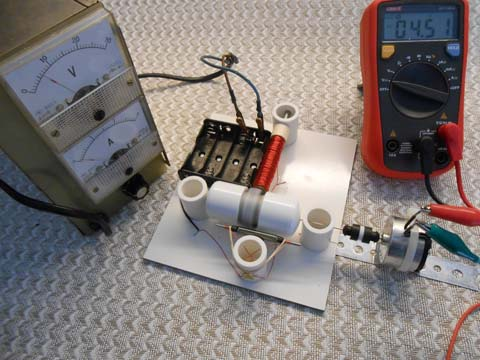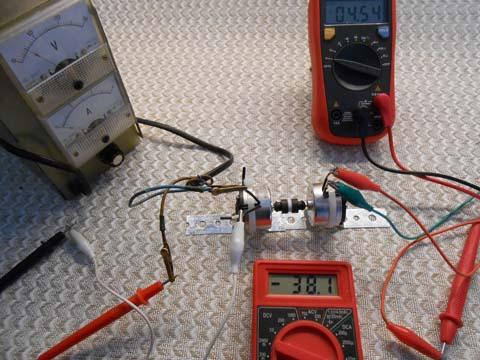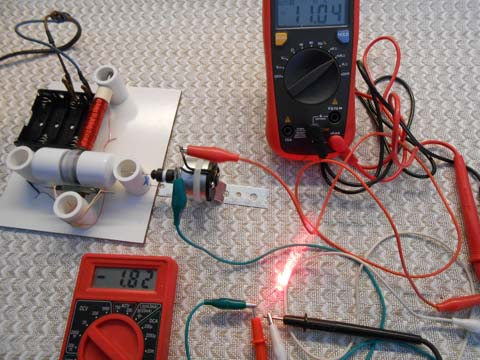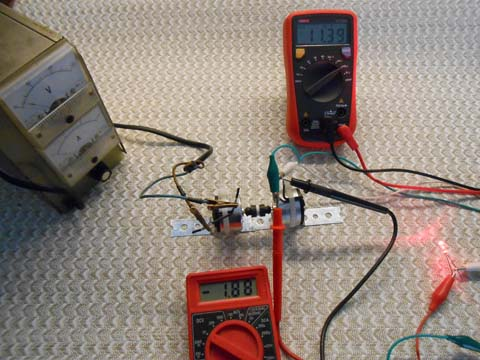Measuring torque is a difficult task. For real dependable measurement you might need special equipment such as an adjustable torque load (it could be small particle brake coupled with the motor shaft) and measuring tool such as dynamometer. That is expensive lab equipment. We came up with relatively simple and inexpensive solution that may be not very accurate but sufficient for science project experiments.

Please see our Calculations section for formulas we used to determine the torque.

Our main idea is based on the fact that torque could be calculated if you know output power and angular speed. It is easy to accurately measure the speed of the motor using measuring tools available in our store, and then convert rotational speed in RPM into angular speed in rad/s.

Output power of the motor for any given speed and torque could be calculated as input power minus losses; or input power x efficiency of the motor.

Input electrical power could be easily calculated by measuring consumed current and voltage and multiplying them. So the only unknown is the efficiency of the motor. How could we estimate it?

Efficiency of the motor is not a number, it is a graph. It could be anything from 0 to maximum efficiency. Usually maximum efficiency is what manufacturers specify for their motors at optimal speed. Small DC motors usually have maximum efficiency of 50-60%.

We decided to estimate the efficiency of the motors built from our kit by comparing them to industrial motors we provide in generator kits. For these motors we have data from manufacturers (see Links). We needed two identical motors so we used two generator kits. We also used a regulated power supply as it provides the ability to accurately adjust input voltage.

In other words we used one motor with known parameters for comparison with our kit motor. We tried to measure and compare how much power these motors needed to do the same work. Second motor worked as a generator where we could vary the load connected to it.

First we connected our motor kit to the generator. At 6 Volts consumed current was 115 mA and generator output was 4.5 Volts. There was no electrical load connected to the generator terminals, only voltmeter for measurements. Motor speed was about 2100 RPM. Power needed to generate this voltage is 0.115 A X 6 V = 0.69 W (Watt).Next we replaced the kit motor with the second generator and adjusted input voltage to achieve the same 4.5 Volts output. In this case the input voltage was 5 Volts and input current was 38 mA. Power needed to generate 4.5 Volts is 0.038 A X 5 V = 0.19 W.To connect the second motor you need to trim one of the bracket flanges with heavy duty scissors. You may use double-sided sticky pad or connect them directly as shown in generator assembly instructions.

As you can see from these measurements to do the same work the kit motor required 3.6 times more energy than industrial one (0.69 / 0.19 = 3.6).

Then we did a series of experiments with increased load when generator was connected to LED.

Here is one of the measurements. The kit motor is connected to generator powering red LED. Input voltage was 4.5 Volts, input current 125 mA. Output voltage was slightly over 1.8 Volts with 11 mA output current which was sufficient to light up the LED. Speed of the motor was about 900 RPM. Input power is 0.125 A X 4.5 V = 0.56 W.Now we replaced the kit motor with the generator and adjusted input voltage to achieve same (or close to it) voltage and current on the LED. Input voltage in this measurement was 2.3 Volts with 42 mA current, so input power was 0.042 A X 2.3 V = 0.097 W.In this measurement in order to provide the same output power the kit motor required 5.8 times more energy than industrial motor.

Be careful in this setup! If you apply bigger voltage the generator may output too much power and burn LED instantly. You may really need a current limiting resistor connected in series with the LED.

In another measurement with the same setup we increased input voltage on the kit motor to 5.5 Volts. Consumed current was 160 mA; output voltage on the LED was 2 V with 26 mA current. For the same output industrial motor needed 3 Volts with 60 mA current. The speed was about 1000 RPM. You may calculate yourself – kit motor required 4.9 times more power.

As a result of these (and many other) measurements we found that kit motor required 3.5-6 times more power than industrial motor to perform identical work; or in other words was 3.5-6 times less efficient. Efficiency was better on higher speeds. As we have power and efficiency curves for the industrial motor from the generator kit and know its maximum efficiency (60% for tested motors) we may roughly estimate maximum efficiency for the kit motor to be about 4 times less than efficiency of tested generator motor, i.e. 15% at 1800-2200 RPM. With generator/LED connected to the motor the speed might be about 1000 RPM and motor efficiency is 10% or even less. These are loose estimates that you can use in your project.

How accurate is this efficiency estimate? It is not very accurate as there are a lot of factors not taken into consideration in this simplified approach. Also we provide the kits, not identical motors. The motor you built may have different efficiency.

If you decide to perform similar experiments for your motor here are some suggestions. First you need to have a power supply with adjustable voltage output. There are many of them available on the Internet or you may build one yourself using for example LM317 chip and few additional parts.

If you want to get more data needed to plot motor efficiency and power curves you may experiment with voltages higher than 6 Volts. In this case the current limiting resistor for the LED is absolutely necessary. Actually you may be better off using variable resistor (sometimes in this setup it is called brake resistor) connected to the generator instead of LED.

In general for small electric motors (and the kit motor is not an exception) maximum power is at approximately 50% of stall torque where the speed usually is at 50% of maximum no-load speed. Maximum efficiency is usually at 10-30% of motor stall torque, or at 70-90% of no-load speed.

Despite the fact that our efficiency and torque calculations may not be very accurate we strongly believe that this should be acceptable for most levels of science projects.

After all it is a great learning experience!

1.•2.